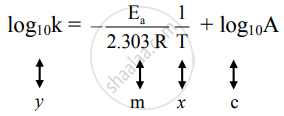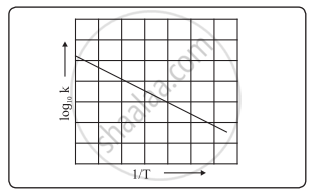# Answer the following in brief. How will you determine activation energy graphically using the Arrhenius equation? - Chemistry

Answer the following in brief.

How will you determine activation energy graphically using the Arrhenius equation?

#### Solution

Graphical representation of activation energy

Arrhenius equation is "k" = "Ae"^((-"E"_"a")/("RT"))

Taking the logarithm of both sides of Eqn. we obtain

In k = -"E"_"a"/"RT" + "In A"

Converting the natural base to base 10 we writeThis equation is of the form of straight-line y = mx + c.

The Arrhenius plot of log10k versus 1/"T" gives a straight line as shown in the diagram. A slope of the line is -"E"_"a"/(2.303 "R") with its intercept being log10A.

From a slope of the line, the activation energy can be determined.Variation of log10k with 1/T

Concept: Temperature Dependence of Reaction Rates
Is there an error in this question or solution?

#### APPEARS IN

Balbharati Chemistry 12th Standard HSC for Maharashtra State Board
Chapter 6 Chemical Kinetics
Exercises | Q 3.14 | Page 137